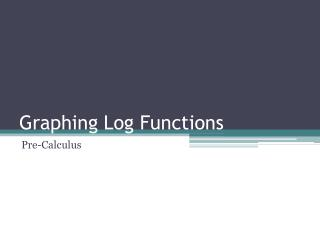DownloadDownload PresentationGraphing Log Functions

# Graphing Log Functions

Télécharger la présentation## Graphing Log Functions

- - - - - - - - - - - - - - - - - - - - - - - - - - - E N D - - - - - - - - - - - - - - - - - - - - - - - - - - -
##### Presentation Transcript

1. Graphing Log Functions Pre-Calculus

2. Graphing Logarithms Objectives: • Make connections between log functions and exponential functions • Construct a table of values for a log function • Graph a log function Vocabulary: logarithms, natural logarithms

3. Graphing Logarithms How do you graph a logarithmic function? Graph f(x) = log3x This is the inverse of g(x) = 3x g(x) = 3x x g(x) x f(x) -2 -1 0 1 2 1/9 1/3 1 3 9 1/9 1/3 1 3 9 -2 -1 0 1 2 f(x) = log3x

4. Steps to Graph a Log Function • Condense your log function. • Rewrite the function as an exponential equation • Make a table for exponential function • Invert values for log function • Plot the points • Connect with a curve

5. Graph the equation: Are there restrictions on the domain and range?

6. Graphing Logarithms Use natural log and ex as rule. Domain of f(x) = ex All reals Range of f(x) = ex Positive reals Domain of f-1(x) = lnx Positive reals Range of f-1(x) = lnx All reals If this works as a general rule… how will the shifting of the exponential or log affect the domain and range?

7. Graph the equation: Domain and Range?

8. Graph the equation: Domain and Range?

9. Homework • P.57-P.58 in Workbook